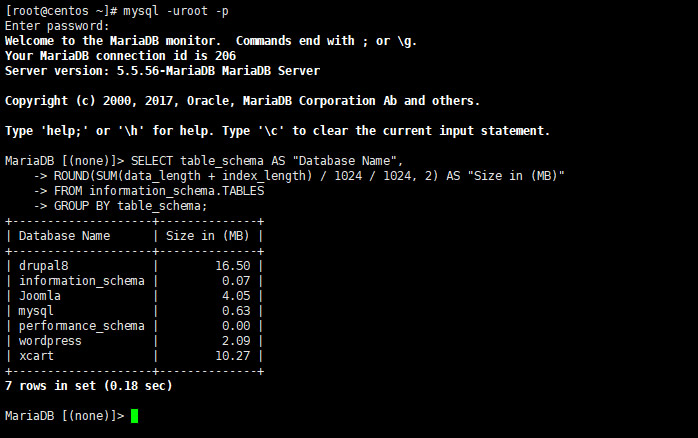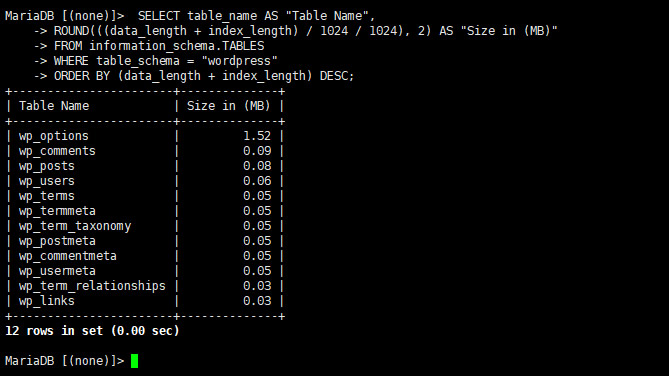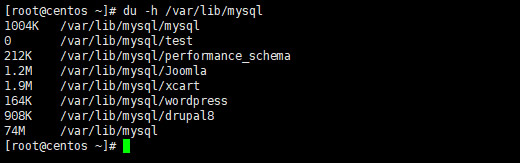```# mysql -u root -p
MariaDB [(none)]> SELECT table_schema AS "Database Name",
ROUND(SUM(data_length + index_length) / 1024 / 1024, 2) AS "Size in (MB)"
FROM information_schema.TABLES
GROUP BY table_schema;``````MariaDB [(none)]> SELECT table_name AS "Table Name",
ROUND(((data_length + index_length) / 1024 / 1024), 2) AS "Size in (MB)"
FROM information_schema.TABLES
WHERE table_schema = "wordpress"
ORDER BY (data_length + index_length) DESC;````# du -h /var/lib/mysql`